Function Repository Resource:

# GraphNeighborhoodVolumes

Find the volumes of successively larger neighborhoods around nodes in a graph

Contributed by: Max Piskunov, Stephen Wolfram and Jan Mangaldan
 ResourceFunction["GraphNeighborhoodVolumes"][graph] gives the numbers of neighbors within successive distances for each vertex in graph. ResourceFunction["GraphNeighborhoodVolumes"][graph,{v1,v2,…}] finds the numbers for the vertices vi. ResourceFunction["GraphNeighborhoodVolumes"][graph,All,r] finds the numbers of neighbors for all vertices out to maximum radius r. ResourceFunction["GraphNeighborhoodVolumes"][graph,vi,Automatic] finds the numbers of neighbors only out to the minimum radius for any of the vertices vi. ResourceFunction["GraphNeighborhoodVolumes"][graph,"Random"→n,…] finds the numbers of neighbors for n randomly chosen vertices.

## Details and Options

ResourceFunction["GraphNeighborhoodVolumes"] works with both directed and undirected graphs. For directed graphs, it takes the volume around a given vertex to be the number of vertices reachable by following directed edges.
ResourceFunction["GraphNeighborhoodVolumes"] accepts the option "Padded", which is False by default. When set to True, ResourceFunction["GraphNeighborhoodVolumes"] finds the numbers of neighbors out to the maximum radius of the graph (or an integer less than the maximum radius) for the specified vertices, padding any vertex of submaximal radius with its final volume value.
ResourceFunction["GraphNeighborhoodVolumes"] uses ParallelMap.

## Examples

### Basic Examples (4)

In a complete graph, the volume around each node includes every node after one step:

 In:=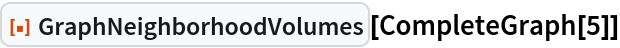Out=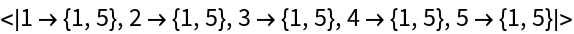In this graph, the volume grows linearly with the radius:

 In:=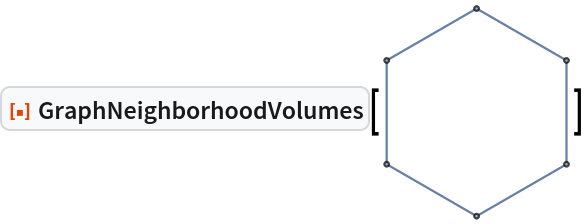Out=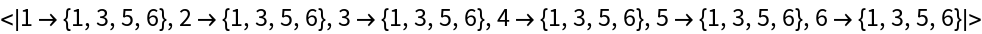Limit to distance 2:

 In:=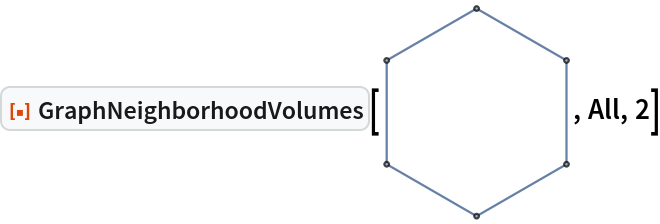Out=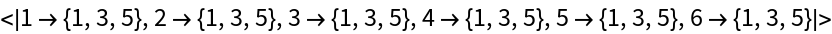In this graph, the volume basically grows exponentially with the radius:

 In:=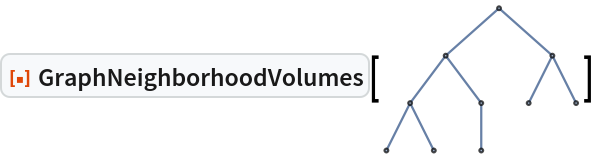Out=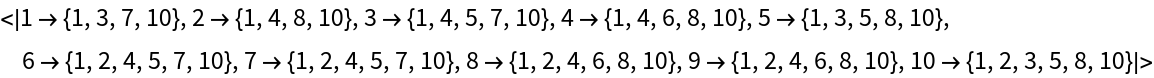Demonstrating the padded volume of the same graph:

 In:=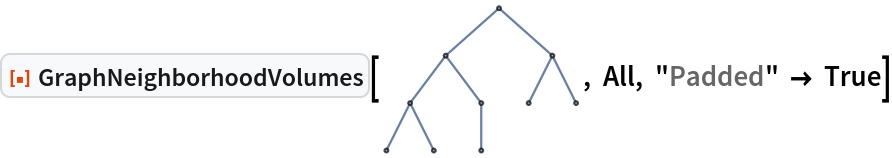Out=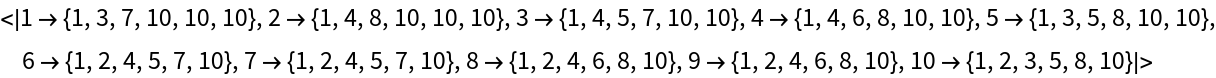The padded volume can also be taken for an integer less than the maximum graph radius:

 In:=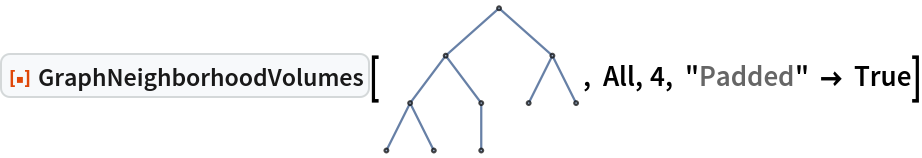Out=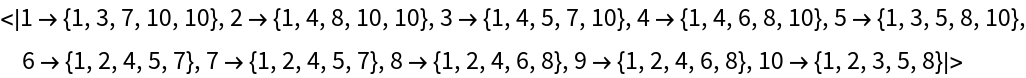Find the numbers of neighbors starting from node 20:

 In:=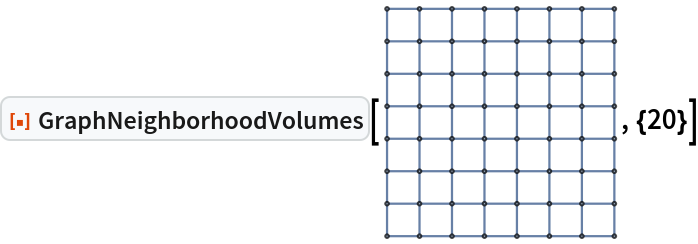Out=### Applications (3)

Find the average volumes of successively larger balls around every vertex:

 In:=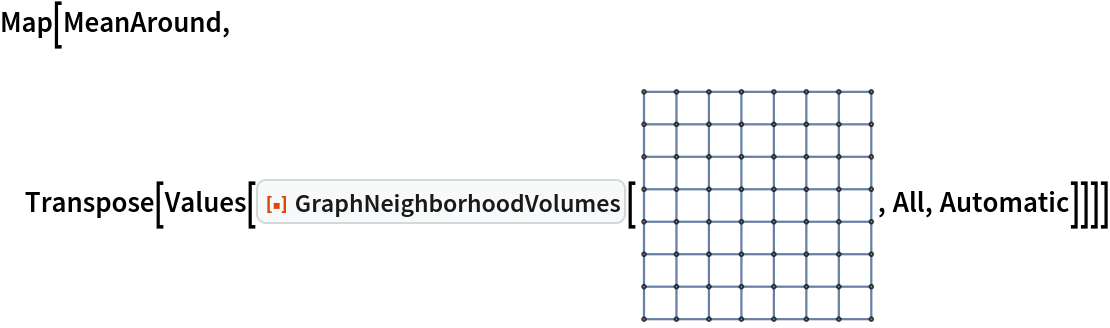Out=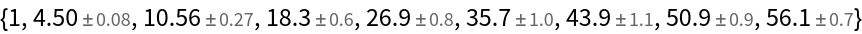Plot the result:

 In:=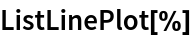Out=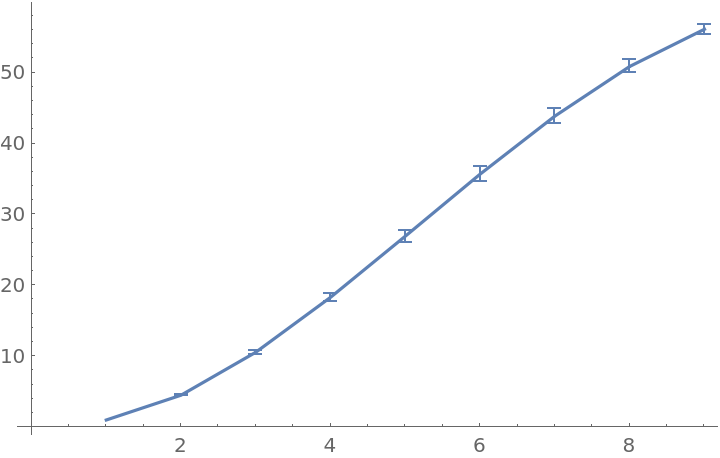Find the average padded volumes of successively larger balls around every vertex:

 In:=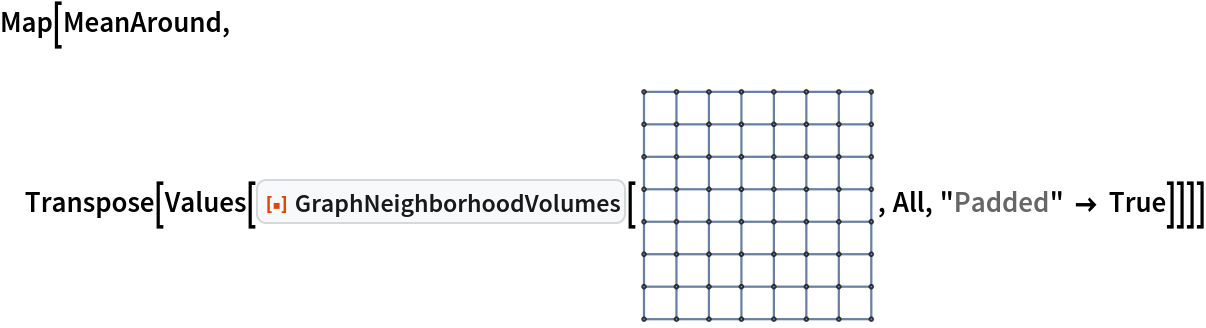Out=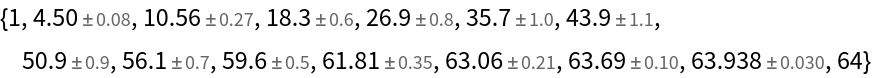In:=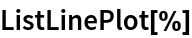Out=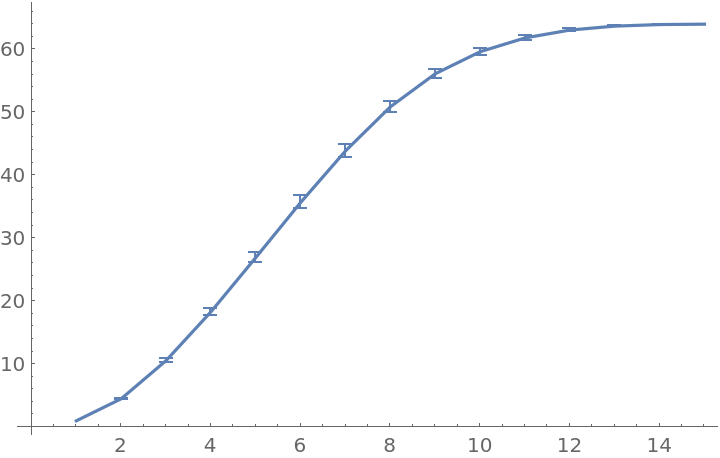Converting the undirected graph from the previous example into a graph with asymmetric edges illustrates how padding helps:

 In:=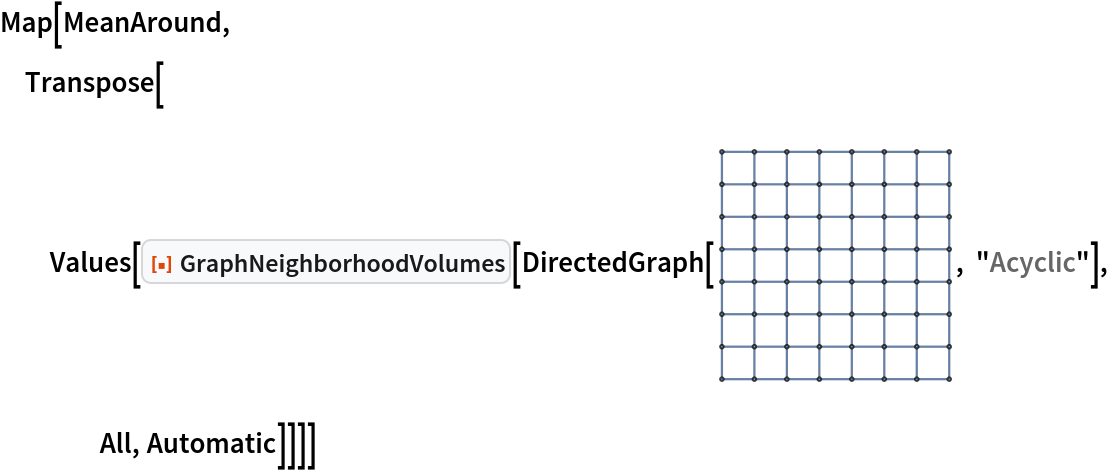Out=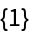Taking the padded graph neighborhood volumes bypasses the limitations in calculating volume that are imposed by vertices in directed graphs that have small radii:

 In:=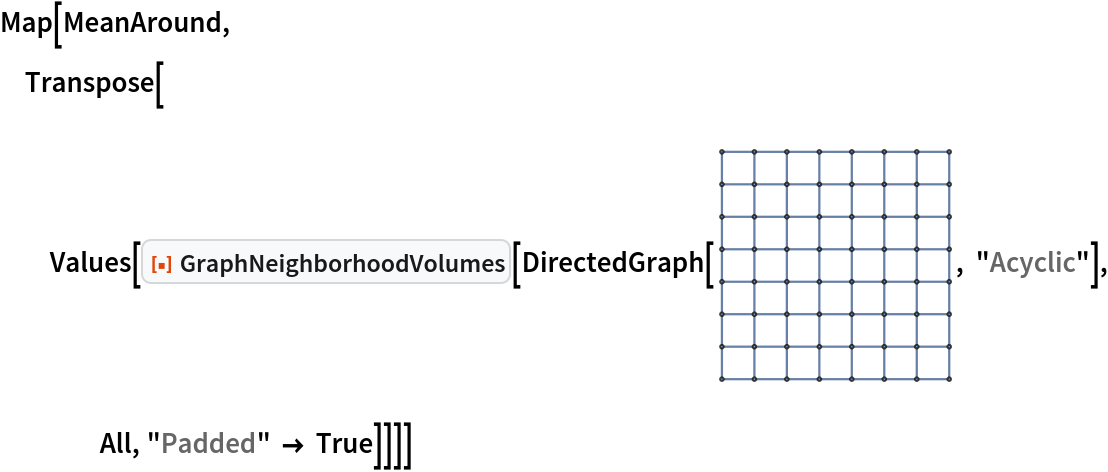Out=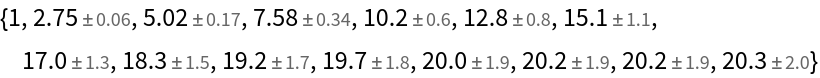In:=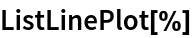Out=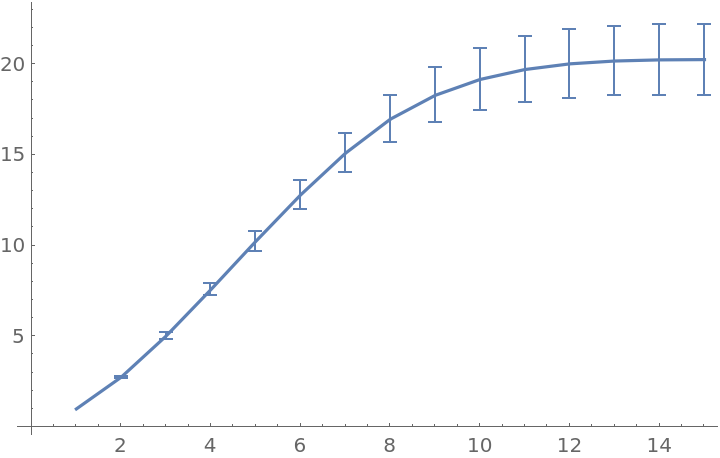## Version History

• 3.0.0 – 15 October 2020
• 2.0.0 – 16 March 2020
• 1.0.0 – 04 December 2019